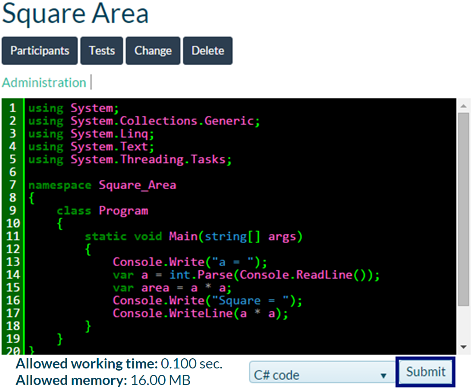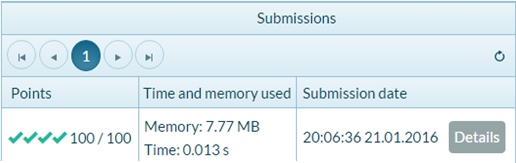# Problem: Calculating Square Area

The first exercise from this topic is the following: write a console program that inputs an integer a and calculates the area of a square with side a. The task is trivial and easy: input a number from the console, multiply it by itself and print the obtained result on the console.

## Hints and Guidelines

We create a new project in the existing Visual Studio solution. In the Solution Explorer right-click on Solution 'Simple-Calculations'. Choose [Add] -> [New Project…]: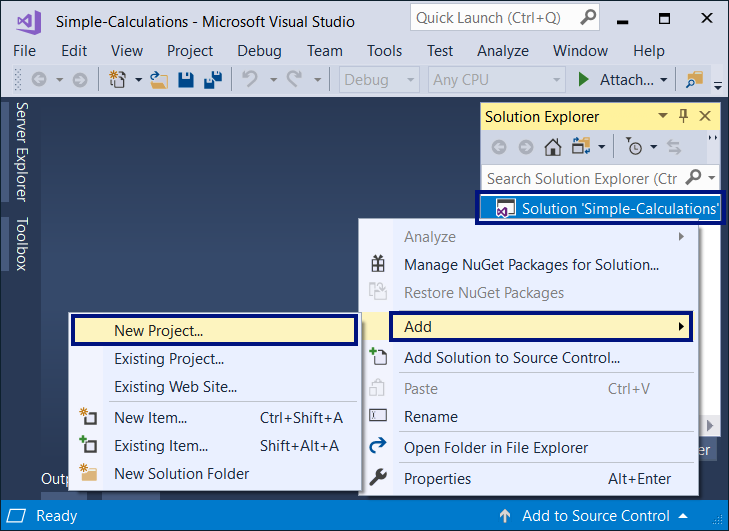A dialogue window is going to be opened for choosing the project type for creation. We choose C# console application with name “Square-Area”: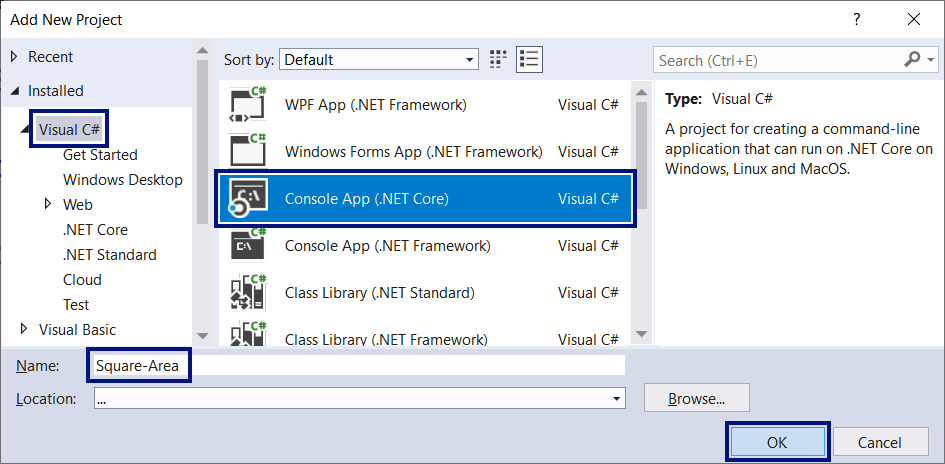We already have a solution with one console application in it. What remains is to write the code for solving this problem. For this purpose, we go to the main method's body Main(string[] args) and write the following code: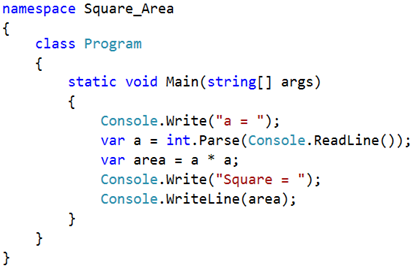The code inputs an integer through a = int.Parse(Console.ReadLine()), afterwards it calculates area = a * a and finally prints the value of the variable area. We start the program with [Ctrl+F5] and test it with different input values: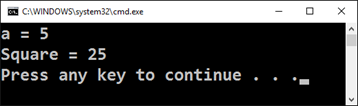## Testing in the Judge System

Test your solution here: https://judge.softuni.org/Contests/Practice/Index/504#0. You have to get 100 points (completely correct solution):